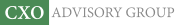Objective research to aid investing decisions

#### Value Investing Strategy (Strategy Overview)

Allocations for December 2023 (Final)
Cash TLT LQD SPY

#### Momentum Investing Strategy (Strategy Overview)

Allocations for December 2023 (Final)
1st ETF 2nd ETF 3rd ETF

# When Potential Upside Is Bigger Than Potential Downside

| | Posted in: Equity Premium

How does the fact that many investments can go up more than they can go down interact with their optimal allocations? In their February 2017 paper entitled “What Our Market Return Forecasts Really Mean: Convexity in Equity Returns and its Implications for Investment Sizing”, Victor Haghani and James White examine how return convexity, return forecast and investment sizing tie together. They measure equity return convexity as arithmetic mean of returns over multiple intervals during a sample period minus geometric mean (compound annual growth rate) over the same sample period. They approximate its value generally as one-half the variance (square of standard deviation) of returns measured across the same multiple intervals. In the context of results from an early 2017 survey of 118 experienced finance professionals about expected U.S. stock market performance, they then consider implications of convexity return when applying the Merton rule for optimal allocation sizing (Sharpe ratio divided by the product of investor risk aversion and standard deviation of returns). Based on mathematical formulas and approximations, they conclude that:

• The convexity return can be material (about 1.3% per year for the U.S. stock market).
• From the survey, average estimated annual stock market real return is 4.2%, with 83% of respondents indicating that their estimates are geometric mean returns.
• Adding U.S. stock market convexity return to the estimated geometric mean return produces an expected arithmetic real return of 5.5%.
• Applying the Merton rule (improperly) to the 4.2% estimate, with moderate investor risk aversion 3, produces equity allocation 55%. Applying it (properly) to the 5.5% estimate produces equity allocation 72%.

In summary, investors may be improperly estimating asset class allocations by using geometric rather than arithmetic mean return forecasts in rule-of-thumb formulas.

Cautions regarding conclusions include:

• There is little support in financial research that investors can accurately forecast stock market returns, and therefore that they can effectively use the Merton rule.
• The mathematics applied in the paper assume a tame (normal) return distribution. To extent that stock market behavior is wild (e.g., its return distribution has fat tails), the formulas do not fit.
• There are no empirical tests in the paper of whether the Merton rule works well.